Related Articles
Replication strategy in Cassandra
• Last Updated : 27 Dec, 2019

In this article, we will discuss Different strategy class options supported by Cassandra such that SimpleStrategy, LocalStrategy, NetworkTopologyStrategy are three Replication strategy in which we generally used Simple and NetworkTopology Strategy in which LocalStrategy is used for system only. let’s discuss one by one.

Different types of Replication strategy class options supported by Cassandra are the following:

```1. SimpleStrategy
2. LocalStrategy
3. NetworkTopologyStrategy ```

These are explained as following below.

1. SimpleStrategy:
It is a simple strategy that is recommended for multiple nodes over multiple racks in a single data center.

Let’s consider taking an example, strategy_demo is a keyspace name in which class is SimpleStrategy and replication_factor is 2 which simply means there are two redundant copies of each row in a single data center. let’s have a look.

Creating a keyspace:

```CREATE KEYSPACE cluster1 WITH
replication = {'class': 'SimpleStrategy',
'replication_factor' : 2}; ```

Let’s verify the keyspace cluster1.

`describe keyspace cluster1; `

Output: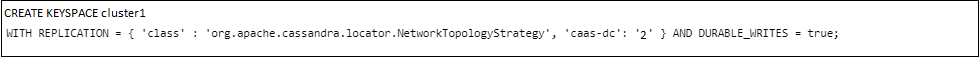2. LocalStrategy:
It is the strategy in which we will use a replication strategy for internal purposes such that is used for system and sys_auth keyspaces are internal keyspaces. In Cassandra internal keyspaces implicitly handled by Cassandra’s storage architecture for managing authorization and authentication.

It is not permissible to creating keyspace with LocalStrategy class if we will try to create such keyspace then it would give an error like “LocalStrategy is for Cassandra’s internal purpose only”.

3. NetworkTopologyStrategy:
It is the strategy in which we can store multiple copies of data on different data centers as per need. This is one important reason to use NetworkTopologyStrategy when multiple replica nodes need to be placed on different data centers.

Let’s consider an example, cluster1 is a keyspace name in which NetworkTopologyStrategy is a replication strategy and there are two data centers one is east with RF( Replication Factor) = 2 and second is west with RF( Replication Factor) = 3.

Let’s have a look.

```CREATE KEYSPACE cluster1 WITH
replication = {'class': 'NetworkTopologyStrategy',
'east' : 2, 'west' : 3}; ```

To verify all internal existing keyspaces used the following CQL query given below.

```select *
from system_schema.keyspaces; ```

Output: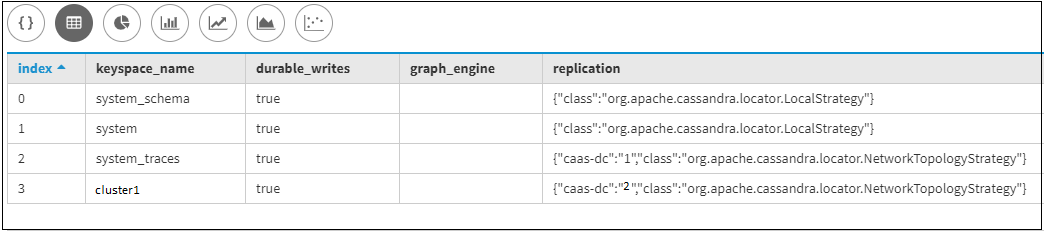```select *
from system_schema.keyspaces
where keyspace_name = 'cluster1'; ```

Output: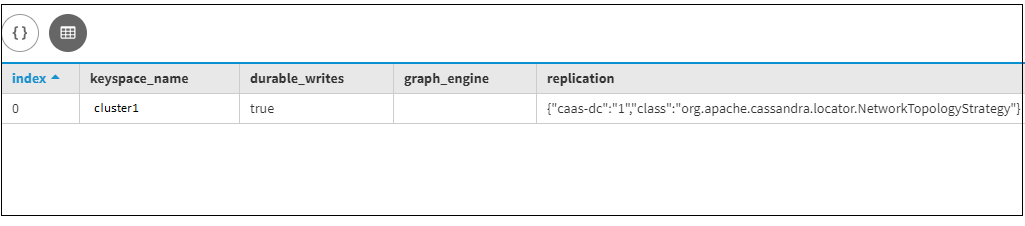To verify all the tables for a specific existing keyspace then used the following CQL query given below.

First, we are going to creating some tables under cluster1 keyspace. let’s have a look.

```create table tb1
(
Id int primary key,
name text,
city text
); ```

Table-2:

```create table tb2
(
Id int primary key,
event text,
team_name text
); ```

Let’s verify the keyspace schema of cluster1 by using the following CQL query.

```SELECT *
FROM system_schema.tables
WHERE keyspace_name = 'cluster1'; ```

Output: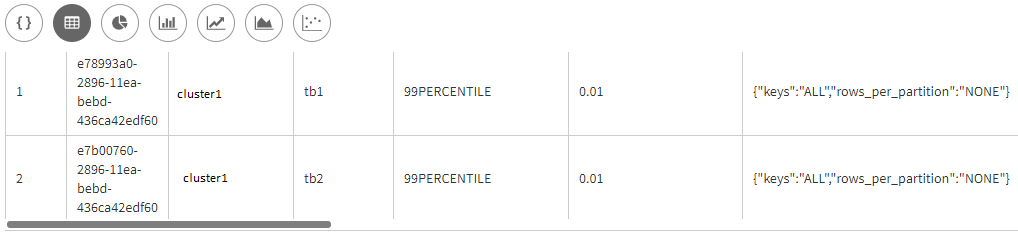To find out all the columns for a specific table with a specific keyspace then used the following CQL query given below.

```SELECT *
FROM system_schema.columns
WHERE keyspace_name = 'cluster1'
AND table_name = 'tb2'; ```

Output: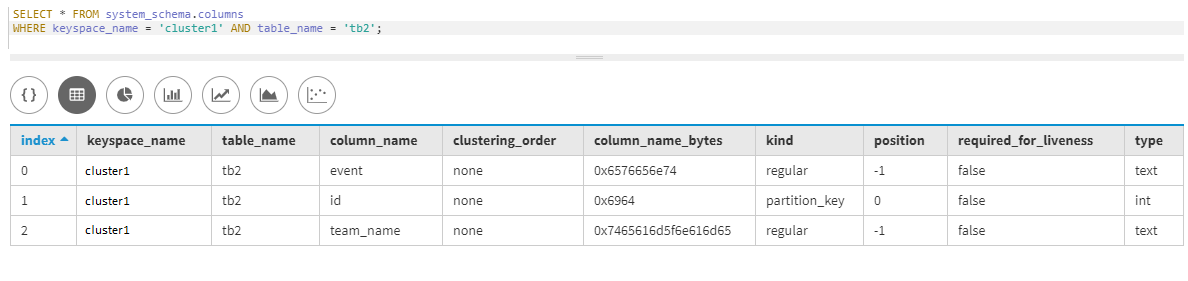System and system_auth Keyspaces:
The system keyspace contains information about available column families, columns, and clusters. The system_auth keyspace mainly contains authentication information, user credentials, and permissions.

My Personal Notes arrow_drop_up